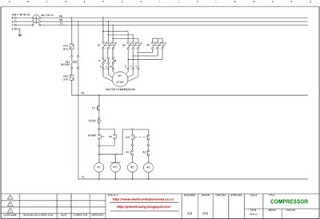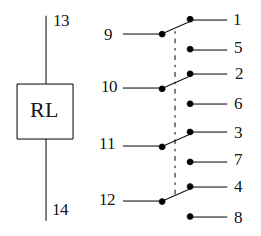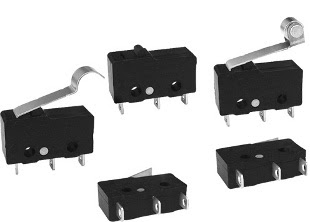## Wiring diagram compressor, star-triangle (star-delta) switching system

In most cases compressor driven by the large power motor, above 10 KW. That used to generate the huge air pressure anyway.

Example of compressor with power 30 KW (40 HP) as shown in the picture below.To reduce inrush current (electric current of early motion) that can reach 200% to 300% of normal current when the motor will rotate, the system needs to be made such a wiring diagram star-triangle (star-delta) switching, as shown by the picture below.Click to enlarge
Electric parts needed for the wiring above:
1. Breaker 100 A 1 pc
2. Transformer step down 380 V / 220 V 3 A 1 pc
3. Magnetic contactor 3P 55 KW coil 220 V 3 pcs
4. Power on delay (Timer) 220 V 1 pc
5. Thermal over load relay 65 A 1 pc
6. Fuse glass 2 A dan 3 A @ 1 pc
7. Start button 1 pc
8. Stop button 1 pc
9. Motor 37 KW 380 V 3 φ 1 unit

## Multiplication and division method of a complex numbers

When the addition and subtraction method of a complex numbers must be in rectangular form, the multiplication and division method of a complex numbers, should be in polar form. Where has the rule: absolute value multiplied (or divided) with absolute value. For angle value have rules: if multiplication, angle value added with angle value and if division, angle value subtracted with angle value.

Sample question 1: (36 ∠ 22°) × (5 ∠ 45°) = ?

Completion:

r (abs) = 36 × 5 = 180

φ (angle) = 22 + 45 = 67

Result:

(36 ∠ 22°) × (5 ∠ 45°) = 180 ∠ 67°

If there is a question multiplication (or division) of rectangular and polar, then the rectangular form must be converted into polar form.

Sample question 2: (14 + j63) ÷ (25 ∠ 37°) = ?

Completion:

Conversion rectangular form (14 + j63) into polar form

14 + j63 = 64.53681 ∠ 77.47119°

we have

r (abs) = 64.53681 ÷ 25 = 2.58147

φ (angle) = 77.47119 - 37 = 40.47119

Result:

(14 + j63) ÷ (25 ∠ 37°) = 2.58147 ∠ 40.47119°

## Addition and subtraction method of a complex numbers

Addition and subtraction method of a complex numbers given by the problem, solved in rectangular form. Where has the rule: real part added (or subtracted) with real part, and imaginary part added (or subtracted) with imaginary part of a complex numbers.

Sample question 1: (30 + j25) + (13 − j5) = ?

Completion:

x (real) = 30 + 13 = 43

y (imaginary) = 25 − 5 = 20

Result:

(30 + j25) + (13 − j5) = 43 + j20

If there is a question addition (or subtraction) of polar and rectangular, then the polar form must be converted into rectangular form.

Sample question 2: (53 + j17) + (21 ∠ 22°) = ?

Completion:

Conversion polar form (21 ∠ 22°) into rectangular form

21 ∠ 22° = 21 (cos 22 + jsin 22) = 19.47086 + j7.86674

we have

x (real) = 53 + 19.47086 = 72.47086

y (imaginary) = 17 + 7.86674 = 24.86674

Result:

(53 + j17) + (21 ∠ 22°) = 72.47086 + j24.86674

## How to operation of a scientific calculator for conversion complex numbers

To solve the problems of a complex numbers (manual method), you should have a scientific calculator. An example Casio S-V.P.A.M fx-570w as I use to my scientific calculator, that looks like the picture below.Operating a scientific calculator you notice calculator buttons present in a [ ] that will be described below, this applies to brands and types of scientific calculator in general.

1. Conversion the complex numbers rectangular form into polar form

Example: 30 + j25 = ?

r (abs) = [ SHIFT ] [ Pol( ] [ 30 ] [ , ] [ 25 ] [ ) ] [ = ] 39.05125

φ (angle) = [ RCL ] [ F ] [ = ] 39.80557

Becomes: 30 + j25 = 39.05125 39.80557°

2. Conversion the complex numbers polar form into rectangular form

Example: 40 ∟ 65° = ?

x (real) = [ SHIFT ] [ Rec( ] [ 40 ] [ , ] [ 65 ] [ ) ] [ = ] 16.90473

y (imaginary) = [ RCL ] [ F ] [ = ] 36.25231

Becomes: 40 65° = 16.90473 + j36.25231

Notes:
• Mode position calculator in D or Deg (degree)
• RCL-E and RCL-F is use to switch the value shown.

## Calculation polar form into rectangular form of a complex numbers

After understanding the manual calculation method to convert complex numbers rectangular form into polar form, this time we'll learn the manual calculation method to convert complex numbers polar form into rectangular form, or the otherwise.

The complex numbers conversion's software, I have given it for free, click Converting complex numbers. It's how to compare the results of manual calculation with the execution of software's program.

Here I will give an example of the manual calculation method to convert complex numbers polar form into rectangular form, with sample questions 40 ∟ 65°

Given the polar form:
40 ∟ 65°
r (abs) = 40
φ (angle) = 65

Completion:
x (real) = r (cos φ) = 40 (cos 65) = 16,90473
y (imaginary) = r (sin φ) = 40 (sin 65) = 36,25231

Then the rectangular form becomes:
x + jy = 16,90473 + j36,25231

See a picture below, the calculation above Polar form into rectangular form

## Calculation rectangular form into polar form of a complex numbers

After learning the complex numbers conversion software (I have given it for free, click Converting complex numbers), then how to compare the results of execution software's program with the manual calculation?

Here I will give an example of the manual calculation method to convert complex numbers rectangular form into polar form, with sample questions 30 + j25

Given the rectangular form:
30 + j25
x (real) = 30
y (imaginary) = 25

Completion:
r (abs) = √(x2 + y2) = √(302 + 252)= 39.05125
φ (angle) = tan-1 (y/x) = tan-1 (25/30) = 39.80557

Then the polar form becomes:
r ∟ φ° = 39.05125 ∟ 39.80557°

See a picture below, the calculation above Rectangular form into polar form

As someone who studied electricity, you will be confused with the imaginary calculations such as: (30 + j25) × (40 ∟ 65°). Two models number in parentheses is a complex numbers, where 30 + j25 is call rectangular form and 40 ∟ 65° is call polar form.

Rectangular form is a number that can be put in the form x + jy, where x and y are real numbers and j is called the imaginary unit. In this expression, x is called the real part and y the imaginary part of the complex number.

Polar form is a number that can be put in the form r ∟ φ°, where r is absolute value and φ is angle value. Together, r and φ give another way of representing complex numbers.

To solve the above calculations problem, you should have a scientific calculator, and you should know how to operate it. Can you do it? And to make it easier I will give complex numbers conversion software, free for you.

## Magnetic relay, TPDT switch and QPDT switch

Magnetic relay or just called relay is a type of switch that is controlled by an electric current. The main part of relay consists of a coil and contacts. Relay coil wrapped around the core. There is an iron armature will be attracted to the core when a current flows through the coil. Armature is mounted on a spring-loaded lever. When the armature attracted to the core, relay contact will change its position from normally closed contacts to the normally open contacts.

A relay can be activated in about 10 ms. Most relay placed in packaging that fully closed, as shown below.Most among the relay has contacts type of SPDT switch, but there are also several types of DPDT switch, TPDT switch (Triple Pole Double Throw) and QPDT switch (Quadruple Pole Double Throw, as shown above right).

The important thing to note on a relay that will be used is the coil voltage and the maximum, current and voltage contacts.

Larger relays can be connected to the currents up to 10 A at a voltage of 250 VAC. The DC maximum voltage for switching is always lower than AC maximum voltage, sometimes even just half of the AC maximum voltage.

Symbol relay with QPDT contact switch## Micro switchMicro term on the micro switch, doesn't mean that the size of micro switch is small. This name indicates that the keys used to operate micro switch, only shifted by a very small distance.

Micro switch is type of very sensitive switch, just a little pressure on a lever may cause the switch move from one position to another. Most micro switches have contacts SPDT type, so that the switch can be used to connect or disconnect, or both simultaneously.

SPDT contacts in the micro switch is generally composed of three terminal tag, such a Common line, NO (Normally Open) contact and NC (Normally Closed) contact. Contacts are equipped with spring-loaded, on normal condition, Common line will be connected to NC contact.

There are various types of micro switch, which can be used according to the applications where the switch should be operated mechanically. Image below shows the various types of micro switches.For example, a micro switch can be mounted in such a way in a refrigerator. Common line and NC contact connected to a lamp. When the door closed, contacts will open and the light will turn off. When the doors opened, the contacts will close and light will turn on.